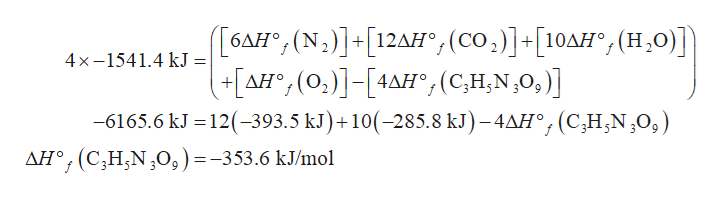# Liquid trinitroglycerin (C3H5N3O9) has been widely used as an explosive and as a medication to relieve angina. The enthalpy of decomposition (ΔHdec) for C3H5N3O9 to form N2(g), CO2(g), H2O(l), and O2(g) is -1541.4 kJ/mol.1. Write the thermochemical equation for the decomposition of 1 mole of trinitroglycerin. 2. Calculate the standard heat of formation (ΔHf) for C3H5N3O9.3. A standard does of C3H5N3O9 for relief of angina is 0.60 mg. If a sample is eventually oxidized completely, how many calories are released?

Question
34 views

Liquid trinitroglycerin (C3H5N3O9) has been widely used as an explosive and as a medication to relieve angina. The enthalpy of decomposition (ΔHdec) for C3H5N3O9 to form N2(g), CO2(g), H2O(l), and O2(g) is -1541.4 kJ/mol.

1. Write the thermochemical equation for the decomposition of 1 mole of trinitroglycerin.

2. Calculate the standard heat of formation (ΔHf) for C3H5N3O9.

3. A standard does of C3H5N3O9 for relief of angina is 0.60 mg. If a sample is eventually oxidized completely, how many calories are released?

check_circle

Step 1

The thermochemical equation for decomposition of 1 mol of trinitroglycerin is shown below:

Step 2

The standard heat of formation is calculated from the balanced equation of decomposition of trinitroglycerin. The balanced equation is shown below:

4C3H5N3O9(l)→6N2(l) + 12CO2(g) + 10H2O(l) + O2(g)

The enthalpy change for the above reaction is 4(-1541.4 kJ). The value of heat o...help_outlineImage Transcriptionclose[6ΔΗ*, (N .)] + [12ΔΗ*, (co]-10ΔΗ*, (Η ,0)] ) ΔΗ, (0, )] -[4ΔΗ', (C,H,N ,O, ] , (0, ] - [4Δ. -6165.6 kJ =12(-393. 5 kJ) + 10(-285.8 kJ) - 4ΔΗ, (CH,N ,0, ) 4x-1541.4 kJ = °;(C;H,N¿0,)] %3D ΔΗ', ( C,Η,N ,Ο, ) - -353.6 kJmol fullscreen

### Want to see the full answer?

See Solution

#### Want to see this answer and more?

Solutions are written by subject experts who are available 24/7. Questions are typically answered within 1 hour.*

See Solution
*Response times may vary by subject and question.
Tagged in

### Chemistry